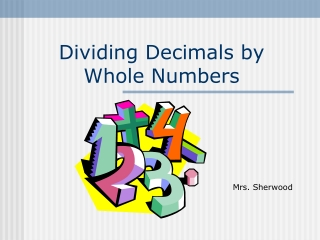DownloadDownload PresentationDividing Decimals by Whole Numbers

# Dividing Decimals by Whole Numbers

Télécharger la présentation## Dividing Decimals by Whole Numbers

- - - - - - - - - - - - - - - - - - - - - - - - - - - E N D - - - - - - - - - - - - - - - - - - - - - - - - - - -
##### Presentation Transcript

1. Dividing Decimals by Whole Numbers Mrs. Sherwood

2. Today we will… • Divide a decimal by a whole number. • Show a remainder as a decimal. • Round a decimal to the nearest tenth.

3. Step 1 Place the decimal point in the quotient above the decimal point in the dividend. .

4. Step 2 Divide as with whole numbers. 6.6 -24 24 24 - 0

6. Now try these two. 42.8 2.61 How did you do?

7. Dividing Whole Numberswith a Decimal Quotient Step 1: Think: Instead of leaving a remainder, add zeros to the dividend and continue to work the problem until your remainder is zero. 6 -120 10

8. Showing the Remainder as a Decimal. Step 2: Place the decimal point in the quotient above the decimal point in the dividend. Add a zero to the dividend. Continue dividing. -120 100 -100

9. Now you try. Hint:You may need to add more than one zero!

10. Check your answers. -36 -8 10 46 -8 -40 20 60 -56 -20 40 -40

11. Let’s try rounding to the nearest 10th. • Look at the number to the right of the number in the 10ths place. • If it is 0, 1, 2, 3, or 4, round down. • If it is 5, 6, 7, 8, or 9, round up. • .925 rounds to .9 .925 .9 3.5 3.467 26.45 26.5 4.01 4 325.23 325.2

12. Great Job!